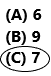# Texas Go Math Grade 1 Lesson 14.2 Answer Key Attributes of Two-Dimensional Shapes

Refer to our Texas Go Math Grade 1 Answer Key Pdf to score good marks in the exams. Test yourself by practicing the problems from Texas Go Math Grade 1 Lesson 14.2 Answer Key Attributes of Two-Dimensional Shapes.

## Texas Go Math Grade 1 Lesson 14.2 Answer Key Attributes of Two-Dimensional Shapes

Explore

Use two-dimensional shapes. Sort them into two groups. Draw to show your work.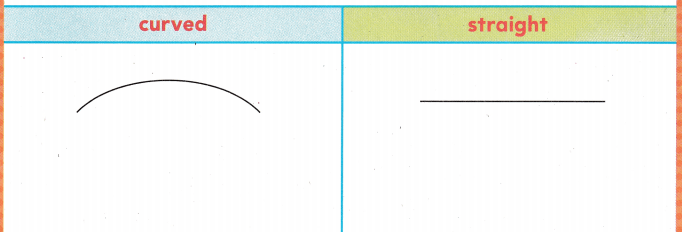Answer: The shapes that are shown below diagram.
Explanation:
CURVED:
* For the curved shapes, there are no sides and corners. Those shapes are called circles, ovals, semi-circles.
STRAIGHT:
* For the straight lines, there will be sides and corners(vertices). Those shapes are called square, rectangle, triangle, trapezium etc.FOR THE TEACHER • Have children sort two-dimensional shapes into groups that are curved and straight. Have them draw the shapes to show how they sorted.
Answer: The groups were sorted below.
Explanation: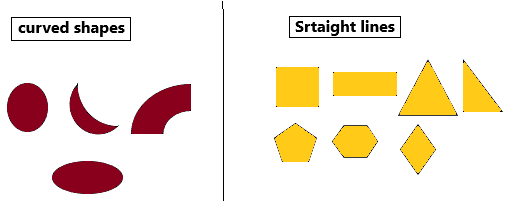The curved shapes are circle, semi-circle, arc, oval.
The straight-line shapes are square, rectangle, triangle, right angle, pentagon, hexagon, rhombus, etc.

Math Talk
Mathematical Processes

Explain how you sorted the shapes into two groups. Name the shapes in each group.
Answer: I sorted the shapes by comparing the sorting rules.Explanation:– Shapes with no sides and no vertices. And it is having a curved shape.Model and Draw

Some shapes have straight sides and vertices.Answer: All the corners and sides are identified in the below diagram.
Explanation: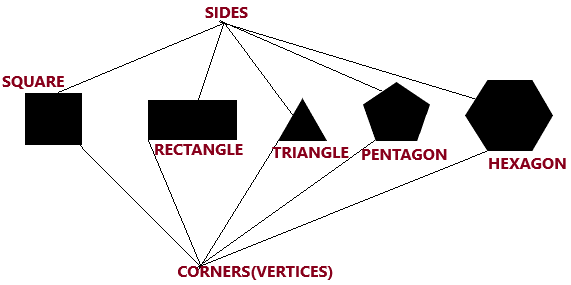Share and Show

Use two-dimensional shapes. Draw and write to complete the chart.

Question 1.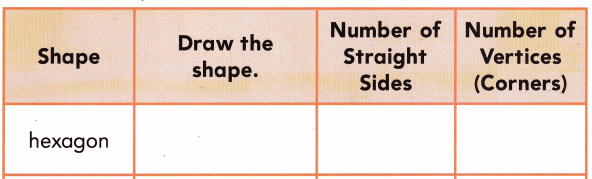Explanation: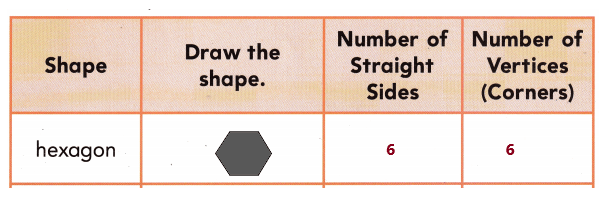Question 2.Explanation:Question 3.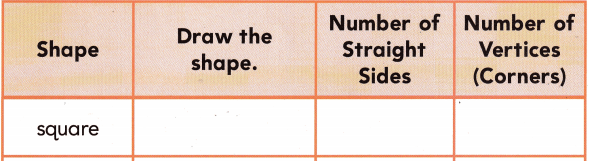Explanation:Question 4.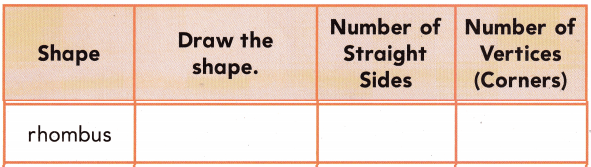Explanation:Question 5.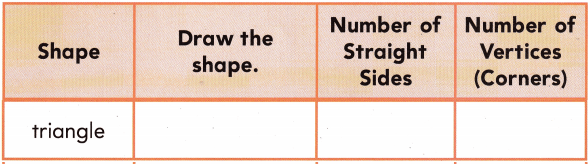Explanation:Problem Solving

Useto trace each straight side.
Useto circle each vertex (corner).
Write the number of sides and vertices (corners).

Question 6.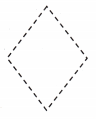_____4____ sides
_____4____ vertices
Explanation:The rhombus is having 4 sides.
The rhombus is having 4 vertices.

Question 7.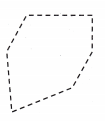_________ sides
_________ vertices
Explanation: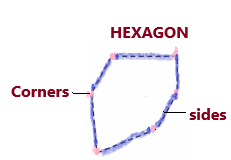There are 6 sides.
There are 6 vertices.

Question 8.
Circle the rhombus.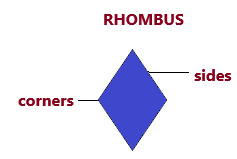There are 4 sides and 4 corners.

Question 9.
Color the hexagon red.There are 6 sides and 6 corners(vertices).

H.O.T. Draw a picture to solve.Question 10.
I am a shape with 3 straight sides and 3 vertices.
Explanation:
Triangle has 3 sides and 3 vertices(corners). These sides and vertices will be shown in the below figure.Question 11.
I am a shape with 4 straight sides that are the same length and 4 vertices.
Explanation:
The square has 4 sides and 4 vertices. All sides are equal.Question 12.
H.O.T. Multi-Step Draw two different shapes that each have only 4 vertices.
Explanation: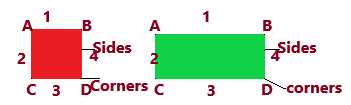Square and rectangle are having 4 corners and 4 sides.

Question 13.
Analyze Tom draws a closed shape with only a curved surface. Which shape does he draw?
(A) circle
(B) hexagon
(C) rectangleExplanation:
In the question, Tom draws a closed shape having with a curved surface which is called the circle.Question 14.
Use words from the box. Write the shape names. Write the number of sides and vertices (corners).
rhombus
rectangle
hexagon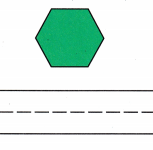__________ sides
__________ vertices
Answer: There are 6 sides .
Hexagon has 6 vertices.
Straight lines are parallel to each other.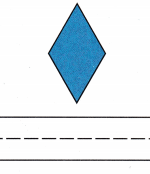__________ sides
__________ vertices
Explanation: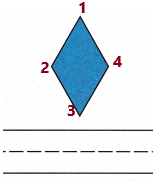Rhombus has 4 sides and 4 vertices.
Rectangle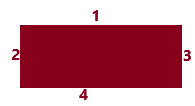Rectangle has 4 sides and 4 vertices.

Question 15.
Texas Test Prep How many vertices does a triangle have?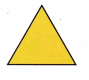(A) 2
(B) 3
(C) 5Explanation:
Triangle has 3 vertices and 3 sides.

TAKE HOME ACTIVITY • Have your child draw a square, a trapezoid, and a triangle. For each shape, have him or her show you the sides and vertices, and tell how many of each.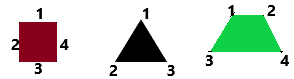Explanation:
The Square has 4 sides and 4 corners.
Triangle has 3 sides and 3 corners.
The trapezoid has 4 sides and 4 corners.

### Texas Go Math Grade 1 Lesson 14.2 Homework and Practice Answer Key

Useto trace each straight side.
Useto circle each vertex (corner).
Write the number of sides and vertices (corners).

Question 1.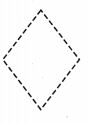__________ sides
__________ verticesThe rhombus has 4 corners and 4 sides.
Blueline represents the sides
Pink dots represent corners(vertices).

Question 2.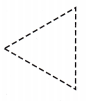__________ sides
__________ vertices
Explanation:Triangle has 3 sides and 3 corners(vertices).

Question 3.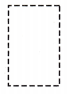__________ sides
__________ verticesRectangle has 4 sides and 4 corners. Opposite sides are equal.

Question 4.__________ sides
__________ verticesHexagon has 6 sides and 6 vertices.

Problem Solving

Draw a picture to solve.

Question 5.
I am a shape with 6 straight sides and 6 vertices.
Explanation:
Hexagon has 6 sides and 6 vertices.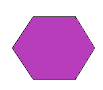Question 6.
I am a shape with 4 straight sides that are not all the same length and 4 vertices.
Explanation:
The square has 4 sides and 4 vertices. All sides are equal.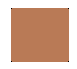Lesson Check

Question 7.
Max draws a shape with 3 straight sides and 3 vertices. Which shape does he draw?
(A) rhombus
(B) triangle
(C) square
Explanation:
Triangle has 3 vertices and 3 sides.Question 8.
Lella draws a shape with 6 straight sides and 6 vertices. Which shape does she draw?
(A) hexagon
(B) rhombus
(C) rectangle
Explanation:
Hexagon has 6 sides and 6 vertices. Lella draws a hexagon shape.Question 9.
How many vertices does a rhombus have?
(A) 6
(B) 4
(C) 3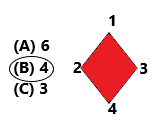Question 10.
Multi-step Sofie has 13 shapes. There are triangles, rectangles, and hexagons. Four shapes have 3 straight sides. Two shapes are hexagons. How many shapes are rectangles?
(A) 6
(B) 9
(C) 7